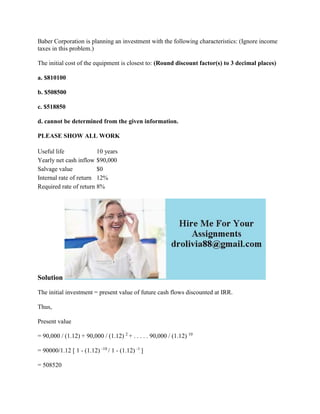Diese Präsentation wurde erfolgreich gemeldet.

# Baber Corporation is planning an investment with the following charact.docx

Anzeige
Anzeige
Anzeige
Anzeige
Anzeige
Anzeige
Anzeige
Anzeige
Anzeige
Anzeige
Anzeige×

1 von 2 Anzeige

# Baber Corporation is planning an investment with the following charact.docx

Baber Corporation is planning an investment with the following characteristics: (Ignore income taxes in this problem.)
The initial cost of the equipment is closest to: (Round discount factor(s) to 3 decimal places)
a. \$810100
b. \$508500
c. \$518850
d. cannot be determined from the given information.
Solution
The initial investment = present value of future cash flows discounted at IRR.
Thus,
Present value
= 90,000 / (1.12) + 90,000 / (1.12) 2 + . . . . . 90,000 / (1.12) 10
= 90000/1.12 [ 1 - (1.12) -10 / 1 - (1.12) -1 ]
= 508520
Thus, initial Investment = 508500 /-
Option B is correct.
Ask if you have doubts. Thanks
.

Baber Corporation is planning an investment with the following characteristics: (Ignore income taxes in this problem.)
The initial cost of the equipment is closest to: (Round discount factor(s) to 3 decimal places)
a. \$810100
b. \$508500
c. \$518850
d. cannot be determined from the given information.
Solution
The initial investment = present value of future cash flows discounted at IRR.
Thus,
Present value
= 90,000 / (1.12) + 90,000 / (1.12) 2 + . . . . . 90,000 / (1.12) 10
= 90000/1.12 [ 1 - (1.12) -10 / 1 - (1.12) -1 ]
= 508520
Thus, initial Investment = 508500 /-
Option B is correct.
Ask if you have doubts. Thanks
.

Anzeige
Anzeige

### Baber Corporation is planning an investment with the following charact.docx

1. 1. Baber Corporation is planning an investment with the following characteristics: (Ignore income taxes in this problem.) The initial cost of the equipment is closest to: (Round discount factor(s) to 3 decimal places) a. \$810100 b. \$508500 c. \$518850 d. cannot be determined from the given information. PLEASE SHOW ALL WORK Useful life 10 years Yearly net cash inflow \$90,000 Salvage value \$0 Internal rate of return 12% Required rate of return 8% Solution The initial investment = present value of future cash flows discounted at IRR. Thus, Present value = 90,000 / (1.12) + 90,000 / (1.12) 2 + . . . . . 90,000 / (1.12) 10 = 90000/1.12 [ 1 - (1.12) -10 / 1 - (1.12) -1 ] = 508520
2. 2. Thus, initial Investment = 508500 /- Option B is correct. Ask if you have doubts. Thanks